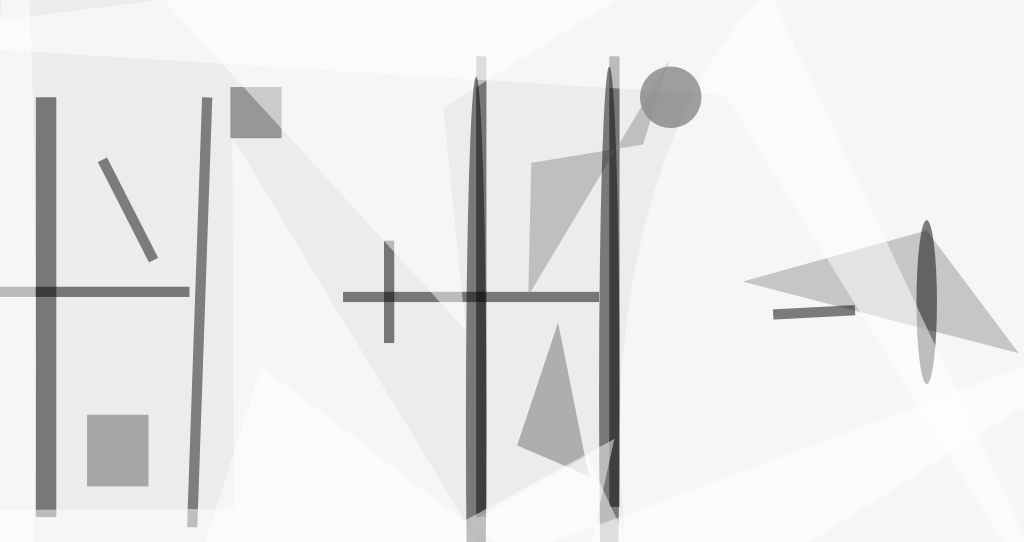A phased-array-fed cylindrical-reflector antenna with superquadric projected aperture has been designed to afford the ability to scan the beam over a wide angular range without having to resort to a large focal-to-diameter ratio and without incurring excessively strong sidelobes. The standard of comparison for this antenna is a paraboloidal-reflector antenna, which offers limited scanning capability at a low value of F/D (where F is focal length and D is diameter). The scanning capability of a paraboloidal-reflector antenna is improved by increasing F/D, but for a given large D, this entails an increase in F and thus in the size of the antenna structure needed to support a feed element at the focal point. The present phased-array-fed cylindrical-reflector antenna would be compact (F/D of only about 0.4, where the cross section of the cylindrical reflector, in x z plane, is a parabola with diameter, D), yet would feature relatively low sidelobes at beam-scan angles as large as 16° off the geometric boresight axis in the plane that contains the cylindrical axis.Figure 1. An Antenna With a Phased-Array Feed and a Parabolic Cylindrical Reflector can be used to reduce sidelobes at large scan angles with suitable choice of projected aperture-shaping parameter, n. The dimensions shown here are only for the numerical example described in the text; the dimensions specific to other applications could differ.

The basic antenna geometry (see Figure 1) is that of a parabolic cylindrical reflector. The cylindrical axis is the y axis, and the geometric boresight axis is the z axis. The phased array of feed elements lies on the focal line, which is parallel to the y axis, at a distance F from the apex of the parabola. The boundary of the reflector and thus of the aperture, as projected onto the x,y plane, is specified by the superquadric curvewhere a and b are the lengths of the x and y semiaxes, respectively, of the aperture; and n is a parameter that can be chosen to control the rounded shape of the corners. The advantage of the superquadric projected aperture is that it is amenable to computational simulation of the performances of antennas with a variety of projected aperture sizes and shapes represented by various combinations of a, b, and n.

Computational simulations have been performed, following diffraction-analysis procedures based on a physical-optics formulation. The geometric parameters used in the simulations were a = 3 m, b = 1.5 m, F = 2.4 m, and three different values of n (2, 4, and 10). The feed elements were positioned at half-wavelength intervals (about 1.07 cm for a frequency of 14 GHz) along the focal line and were polarized along the y axis. The amplitudes of the array-feed excitations were tapered symmetrically about the central feed element, in proportion to [cos(πy/D1)]2, where D1 is the length of the array feed. The beam scan was realized through phase progression of the feeds.Figure 2. Some Results of Numerical Simulations are plotted for three different scan angles in the full-illumination case. In these plots, "elevation" signifies the far-field angle off the z axis in the y,zplane, and "azimuth" signifies the far-field angle off the z axis in the plane that includes x axis and the beam peak for the given scan angle.

For each value of n, two cases were considered. In one case, the reflector was under-illuminated: 233 feed elements were disposed symmetrically along the focal line from y = -1.25 to y = 1.25 m. In the other case, the reflector was fully illuminated: 281 feed elements were symmetrically disposed along the focal line for the full length of the cylindrical axis from y= -1.5 to y = 1.5 m.

The results of the computational simulation showed, among other things, that for a given beam scan off the z axis in elevation, the sidelobes are higher in the full-illumination than in the under-illumination case. The results also showed that the sidelobe levels for a given beam scan can be monotonically reduced at far angle from the peak of the beam by suitable choice of n, and the impact of the shaping parameter, n, on the sidelobe levels is more pronounced as the beam is scanned away from the boresight axis of the antenna (see Figure 2).

This work was done by Ziad Hussein and Eastwood Im of Caltech for NASA's Jet Propulsion Laboratory.

NPO-20494##### Electronics Tech Briefs Magazine

This article first appeared in the February, 2000 issue of Electronics Tech Briefs Magazine.

Read more articles from the archives here.